# 12.6 - Correlation & Regression Example

12.6 - Correlation & Regression Example

Each spring in Indianapolis, IN the National Football League (NFL) holds its annual combine. The combine is a place for college football players to perform various athletic and psycholological tests in front of NFL scouts.

Two of the most recognized tests are the forty-yard dash and the vertical jump. The forty-yard dash is the most popular test of speed, while the vertical jump measures the lower body power of the athlete.

Football players train extensively to improve these tests. We want to determine if the two tests are correlated in some way. If an athlete is good at one will they be good at the other? In this particular example, we are going to determine if the vertical jump could be used to predict the forty-yard dash time in college football players at the NFL combine.

Data from the past few years were collected from a sample of 337 college football players who performed both the forty-yard dash and the vertical jump.

#### Solution

Correlation
##### Is there an association?

The correlation is given in the Minitab output:

Pearson correlation for Forty and Vertical = -0.729589

P-Value = <0.0001

The variables have a strong, negative association.

Simple Linear Regression
##### Can we predict 40-yd dash time from vertical jump height?

Next, we'll conduct the simple linear regression procedure to determine if our explanatory variable (vertical jump height) can be used to predict the response variable (40 yd time).

##### Assumptions

First, we'll check our assumptions to use simple linear regression.

Assumption 1: Linearity

The scatterplot below shows a linear, moderately negative relationship between the vertical jump (in inches) and the forty-yard dash time (in seconds).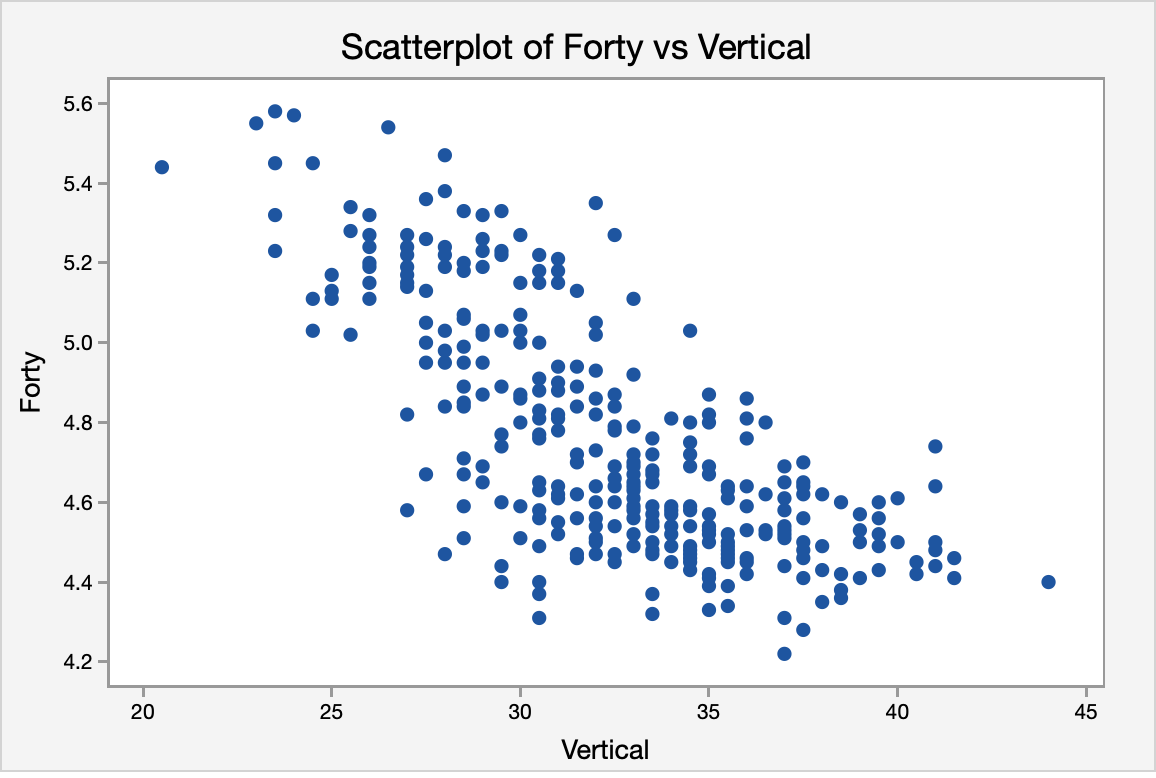Assumption 2: Independence of Errors

The correlation shown in the Versus Fits scatterplot is approximately 0. This assumption is met.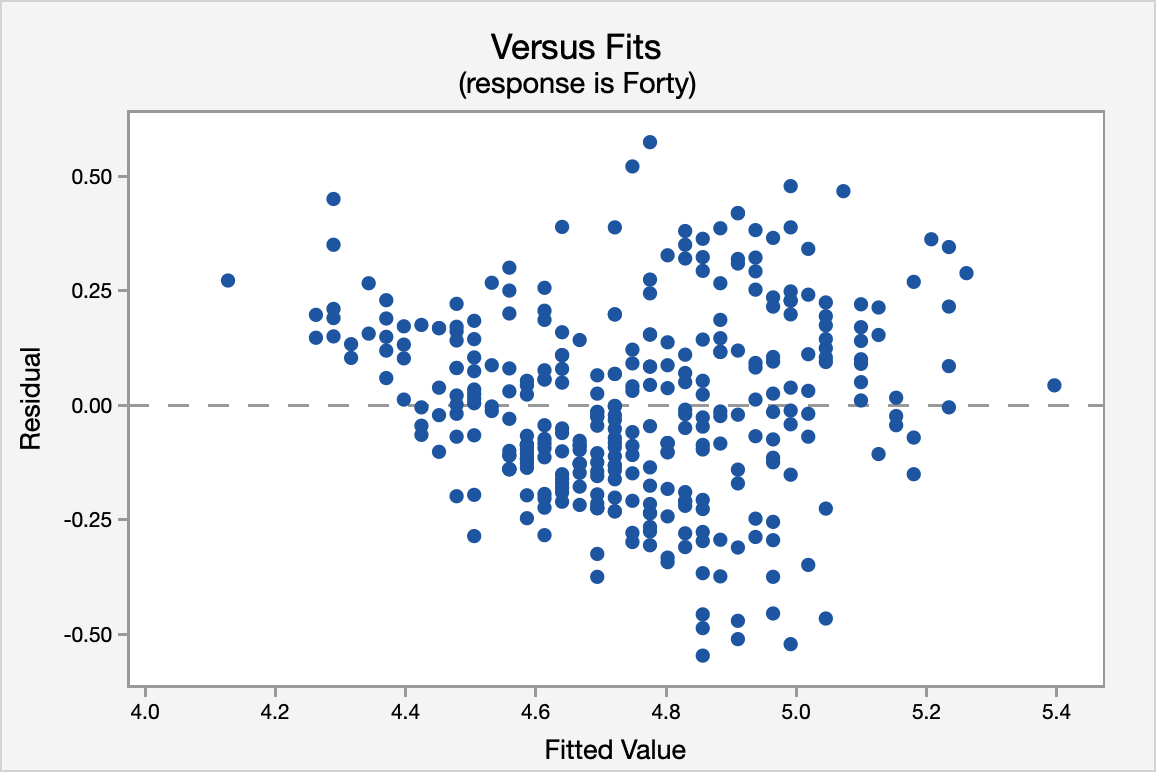Assumption 3: Normality of Errors

The normal probability plot and the histogram of the residuals confirm that the distribution of residuals is approximately normal.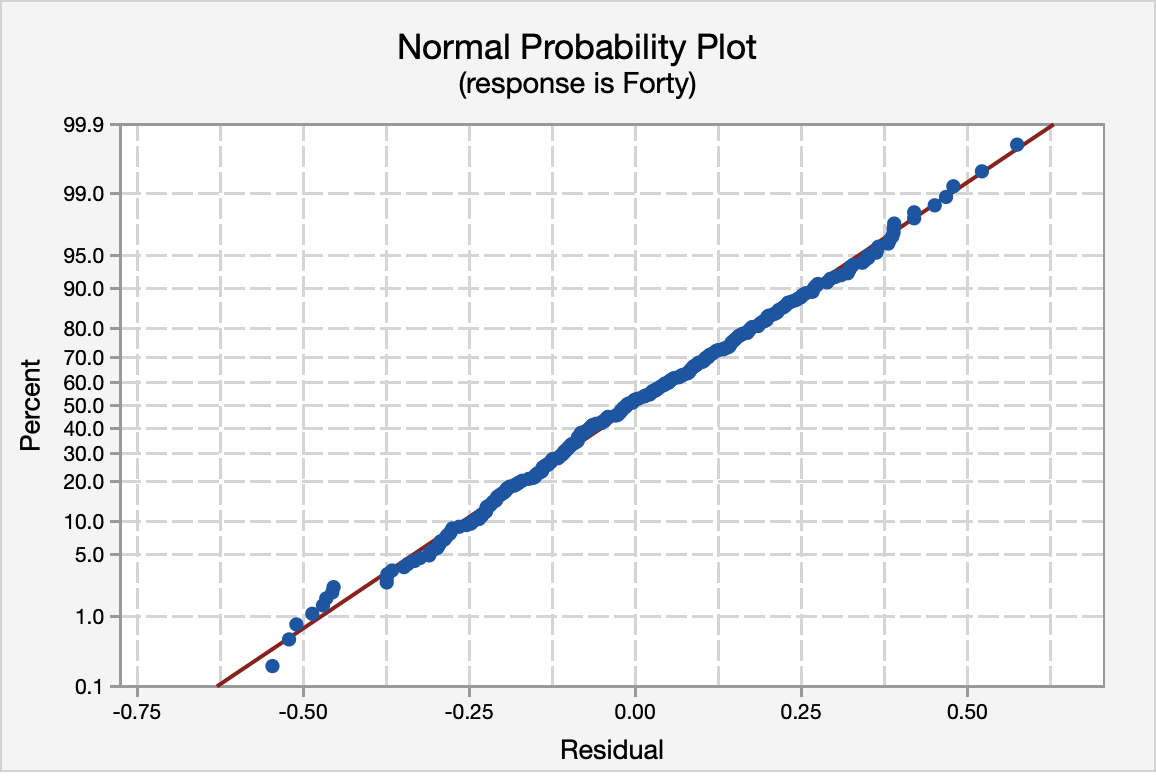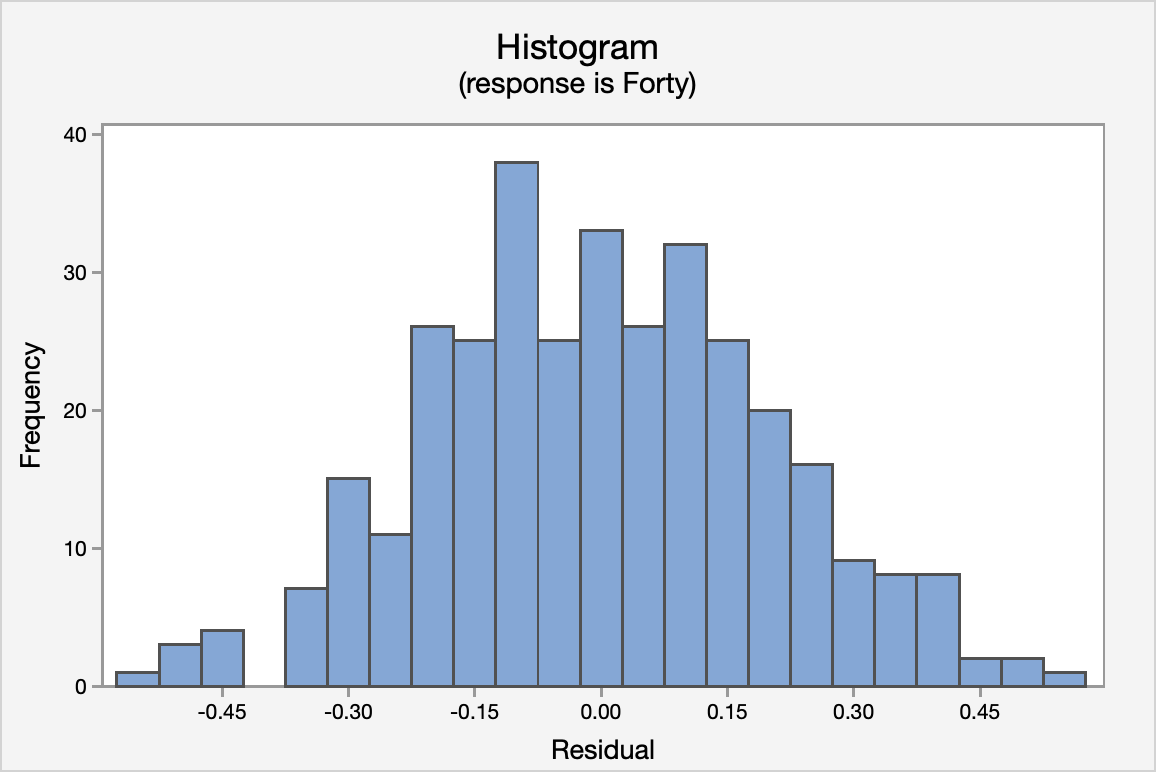Assumption 4: Equal Variances

Again, using the Versus Fits scatterplot we see no pattern among the residuals. We can assume equal variances.##### ANOVA Table

The ANOVA source table gives us information about the entire model. The $$p$$ value for the model is <0.0001. Because this is simple linear regression (SLR), this is the same $$p$$ value that we found earlier when we examined the correlation and the same $$p$$ value that we see below in the test of the statistical significance for the slope. Our $$R^2$$ value is 0.5323 which tells us that 53.23% of the variance in forty-yard dash times can be explained by the vertical jump height of the athlete. This is a fairly good $$R^2$$ for SLR.

##### Analysis of Variance
Regression 1 15.8872 15.8872 381.27 <0.0001
Error 335 13.9591 0.0417
Total 336 29.8464
##### Model Summary
0.204130 53.23% 53.09%
##### Coefficients
Predictor Coef SE Coef T-Value P-Value
Constant 6.50316 0.09049 71.87 <0.0001
Vertical -0.053996 0.002765 -19.53 <0.0001
##### Regression Equation
The regression equation for the two variables is:

Forty = 6.50316 - 0.053996 Vertical

The regression equation indicates that for every inch gain in vertical jump height the 40-yd dash time will decrease by 0.053996 (the slope of the regression line). Finally, the fitted line plot shows us the regression equation on the scatter plot.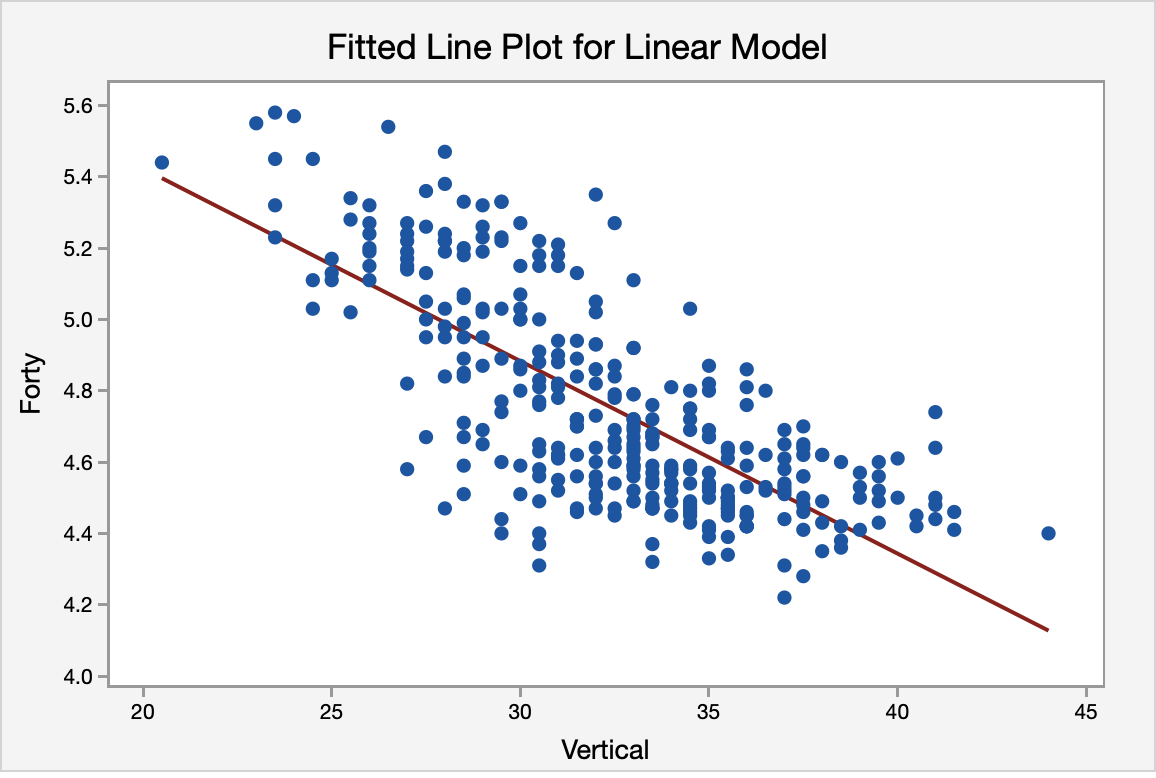Link ↥ Has Tooltip/Popover Toggleable Visibility